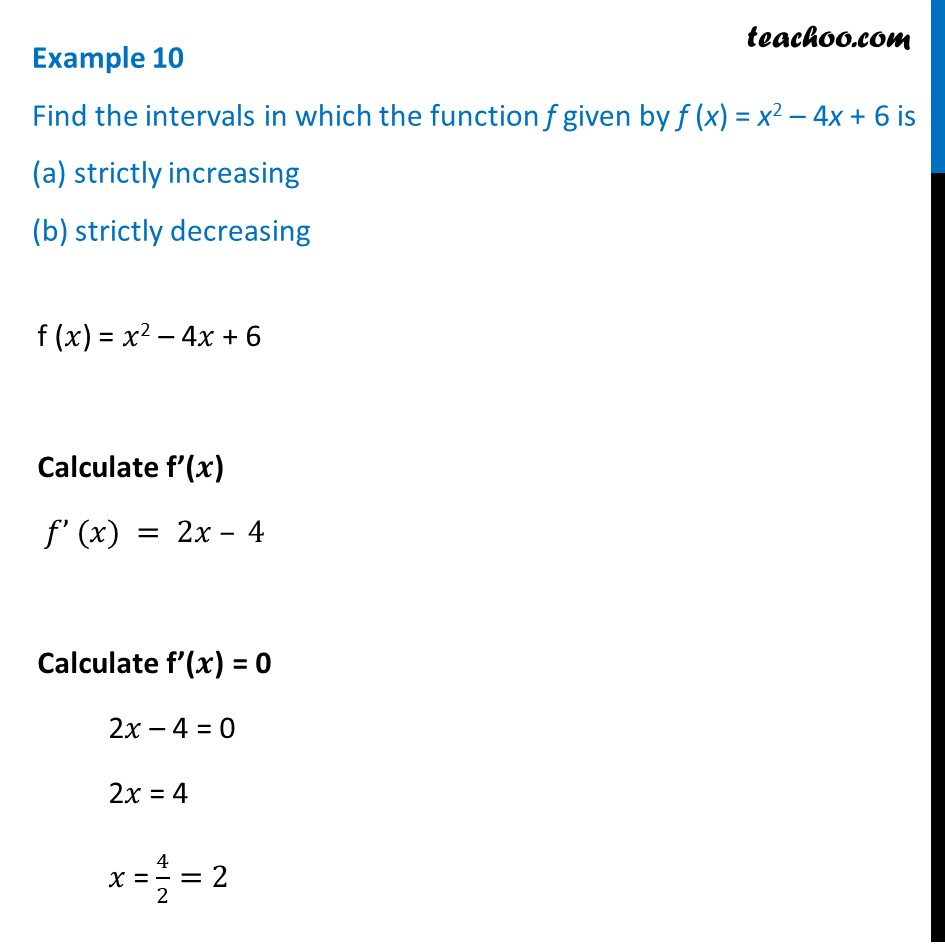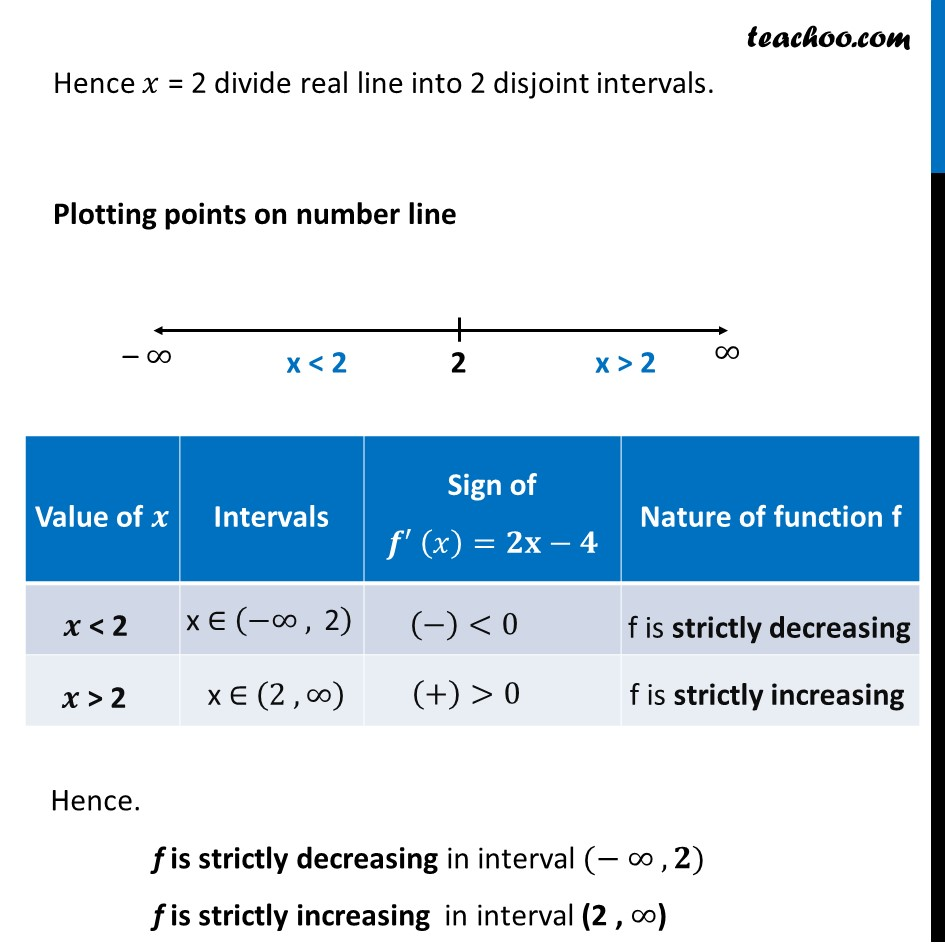Examples

Chapter 6 Class 12 Application of Derivatives
Serial order wiseLearn in your speed, with individual attention - Teachoo Maths 1-on-1 Class

### Transcript

Example 10 Find the intervals in which the function f given by f (x) = x2 – 4x + 6 is (a) strictly increasing (b) strictly decreasingf (𝑥) = 𝑥2 – 4𝑥 + 6 Calculate f’(𝒙) 𝑓’ (𝑥) = 2𝑥 – 4 Calculate f’(𝒙) = 0 2𝑥 – 4 = 0 2𝑥 = 4 𝑥 = 4/2=2 Hence 𝑥 = 2 divide real line into 2 disjoint intervals. Plotting points on number line